## 一、二次函数概念

• 1
二次函数的概念一般地，形如y=ax²+bx+c（a，b，c是常数，a≠0）的函数，叫做二次函数。

这里需要强调：和一元二次方程类似，二次项系数a≠0，而b，c可以为零．二次函数的定义域是全体实数．

• 2
二次函数y=ax²+bx+c的结构特征：
⑴ 等号左边是函数，右边是关于自变量x的二次式，x的最高次数是2． ⑵ a，b，c是常数，a是二次项系数，b是一次项系数，c是常数项．
END

## 二、二次函数的基本形式

• 1
二次函数基本形式：y=ax²的性质：
a的绝对值越大，抛物线的开口越小。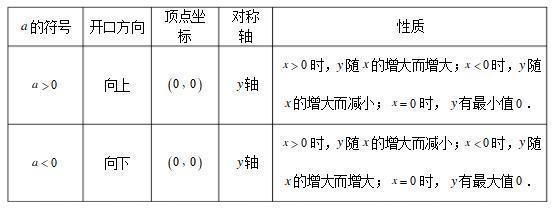• 2
y=ax²+c的性质：（上加下减）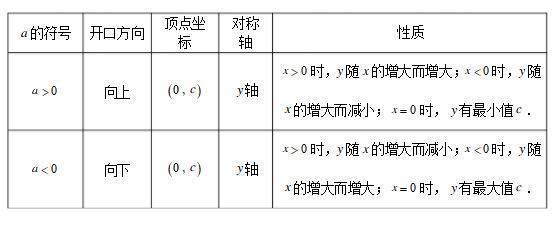• 3
y=a(x-h)²的性质：(左加右减）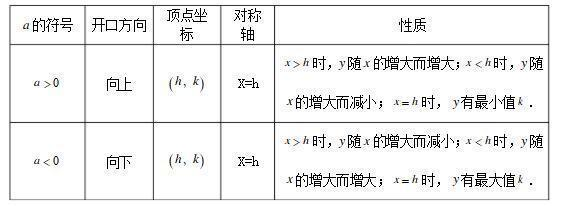• 4
y=a(x-h)²+k的性质：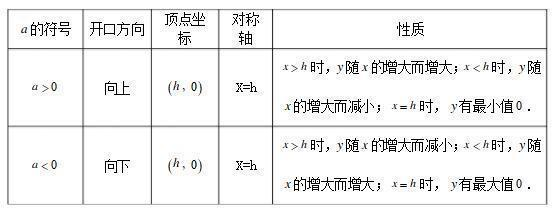END

## 三、二次函数图象的平移

• 1
方法一：
平移步骤：

⑴将抛物线解析式转化成顶点式y=a(x-h)²+k，确定其顶点坐标(h,k)；⑵保持抛物线y=ax²的形状不变，将其顶点平移到(h,k)处，具体平移方法如下：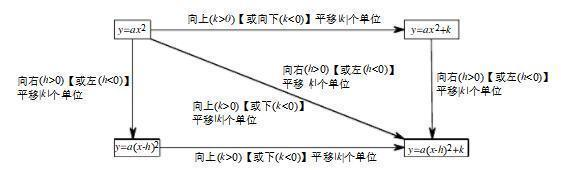• 2
平移规律：
在原有函数的基础上“h值正右移，负左移；k值正上移，负下移”．概括成八个字“左加右减，上加下减”．
• 3
方法一：
(1)y=ax²+bx+c沿y轴平移:向上（下）平移m个单位，y=ax²+bx+c变成y=ax²+bx+c+m
（或y=ax²+bx+c-m）
(2)y=ax²+bx+c沿y轴平移:向左（右下）平移m个单位，y=ax²+bx+c变成y=a(x+m)²+b(x+m)+c
（或y=a(x-m)²+b(x-m)+c）
END